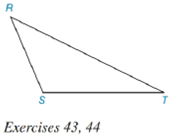Chapter 7.1, Problem 42E### Elementary Geometry for College St...

6th Edition
Daniel C. Alexander + 1 other
ISBN: 9781285195698

#### Solutions

Chapter
Section### Elementary Geometry for College St...

6th Edition
Daniel C. Alexander + 1 other
ISBN: 9781285195698
Textbook Problem
1 views

# Note: Exercises preceded by an asterisk are of a more challenging nature.Construct the three perpendicular bisectors of sides and then the circumscribed circle for obtuse ∆ R S T .To determine

To construct:

Three perpendicular bisectors of sides and then the circumscribed circle for obtuse RST.

Explanation

Procedure:

1. Construct an obtuse triangle RST.

2. Draw perpendicular bisectors of the three sides.

3. All the three bisectors meet at a point O

### Still sussing out bartleby?

Check out a sample textbook solution.

See a sample solution

#### The Solution to Your Study Problems

Bartleby provides explanations to thousands of textbook problems written by our experts, many with advanced degrees!

Get Started

#### 4. If total costs are and total revenues Are ,find the break-even points.

Mathematical Applications for the Management, Life, and Social Sciences

#### Find an equation for the conic whose graph is shown. 7.

Precalculus: Mathematics for Calculus (Standalone Book)

#### limxx2+13x+2= a) 0 b) 1 c) 13 d) does not exist

Study Guide for Stewart's Single Variable Calculus: Early Transcendentals, 8th

#### Simplify. (3x+xy6)+18+(x+xy)

Mathematics For Machine Technology